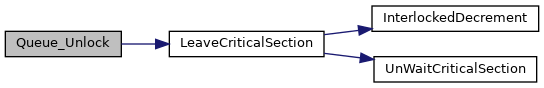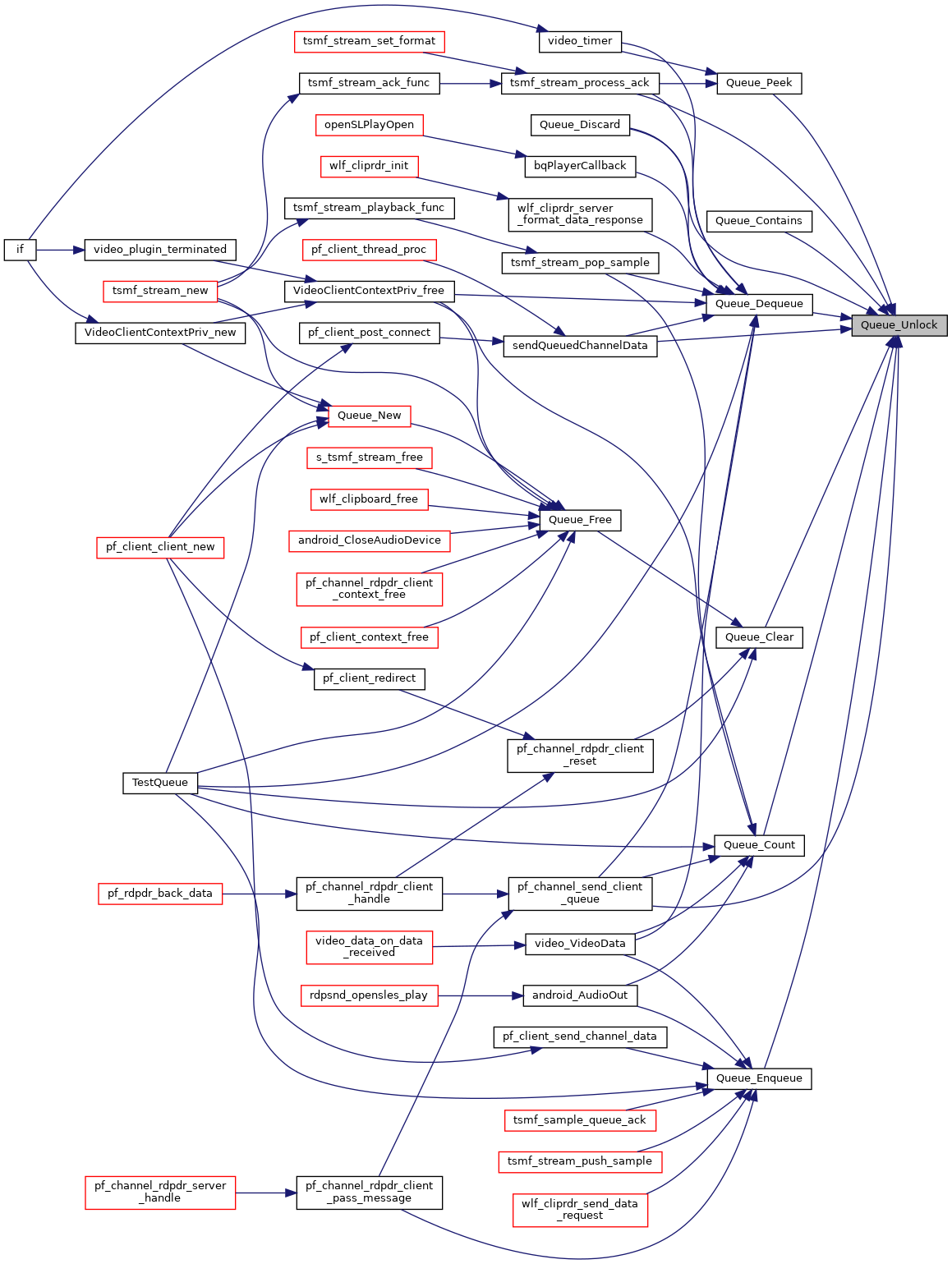FreeRDP
Queue.c File Reference
`#include <winpr/config.h>`
`#include <winpr/crt.h>`
`#include <winpr/assert.h>`
`#include <winpr/collections.h>`

## Functions

size_t Queue_Count (wQueue *queue)

void Queue_Lock (wQueue *queue)

void Queue_Unlock (wQueue *queue)

HANDLE Queue_Event (wQueue *queue)

wObjectQueue_Object (wQueue *queue)

void Queue_Clear (wQueue *queue)

BOOL Queue_Contains (wQueue *queue, const void *obj)

static BOOL Queue_EnsureCapacity (wQueue *queue, size_t count)

BOOL Queue_Enqueue (wQueue *queue, const void *obj)
Pushes a new element into the queue. If a fnObjectNew is set, the element is copied and the queue takes ownership of the memory, otherwise the ownership stays with the caller. More...

void * Queue_Dequeue (wQueue *queue)
returns the element at the top of the queue. The element is removed from the queue, ownership of the element is passed on to the caller. More...

void * Queue_Peek (wQueue *queue)
returns the element at the top of the queue. The element is not removed from the queue, ownership of the element stays with the queue. More...

Removes the element at the top of the queue. If fnObjectFree is set, the element is freed. This can be used in combination with Queue_Peek to handle an element and discard it with this function afterward. An alternative is Queue_Dequeue with calling the appropriate free function afterward. More...

static BOOL default_queue_equals (const void *obj1, const void *obj2)

wQueue * Queue_New (BOOL synchronized, SSIZE_T capacity, SSIZE_T growthFactor)

void Queue_Free (wQueue *queue)

## ◆ default_queue_equals()

 static BOOL default_queue_equals ( const void * obj1, const void * obj2 )
static
Here is the caller graph for this function: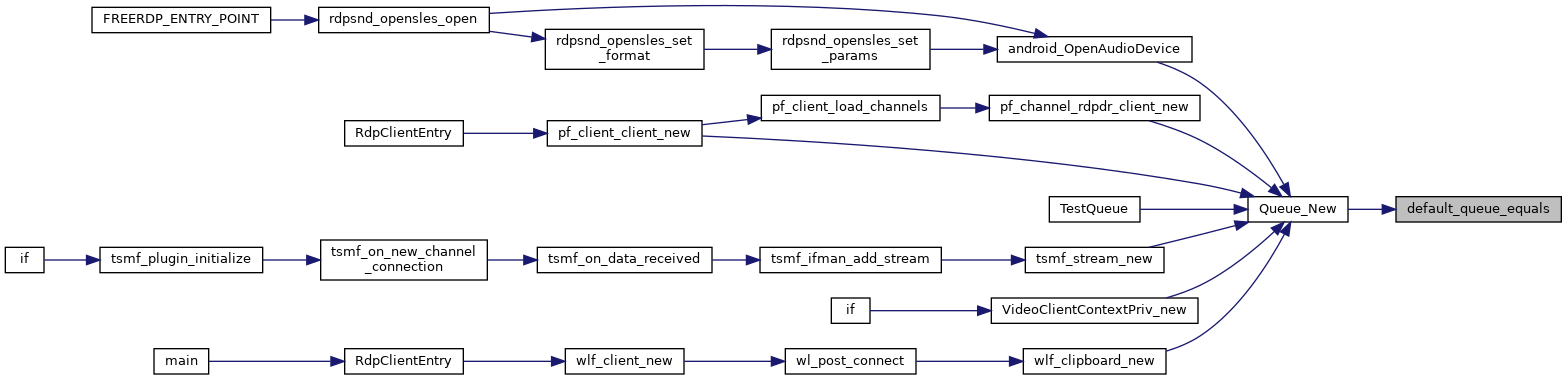## ◆ Queue_Clear()

 void Queue_Clear ( wQueue * queue )

Methods Removes all objects from the Queue.

Here is the call graph for this function: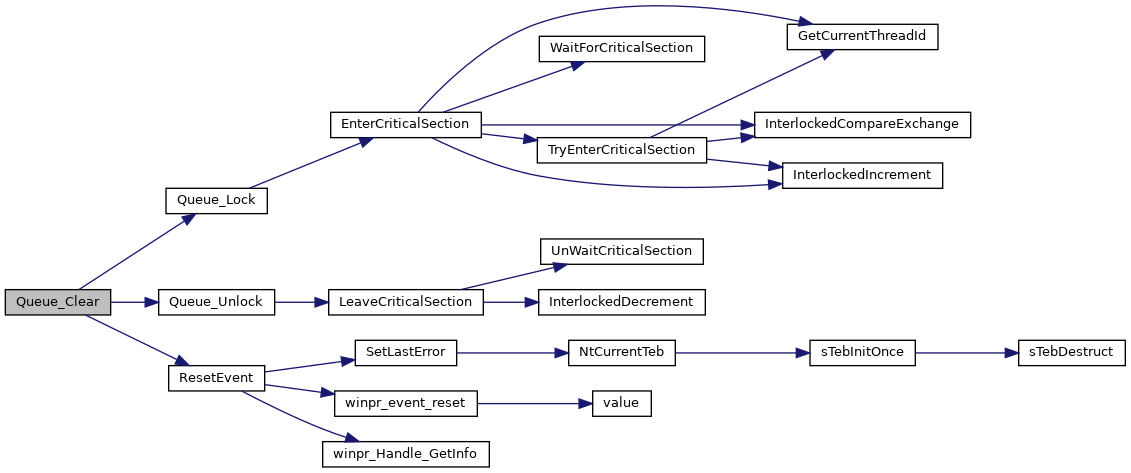Here is the caller graph for this function: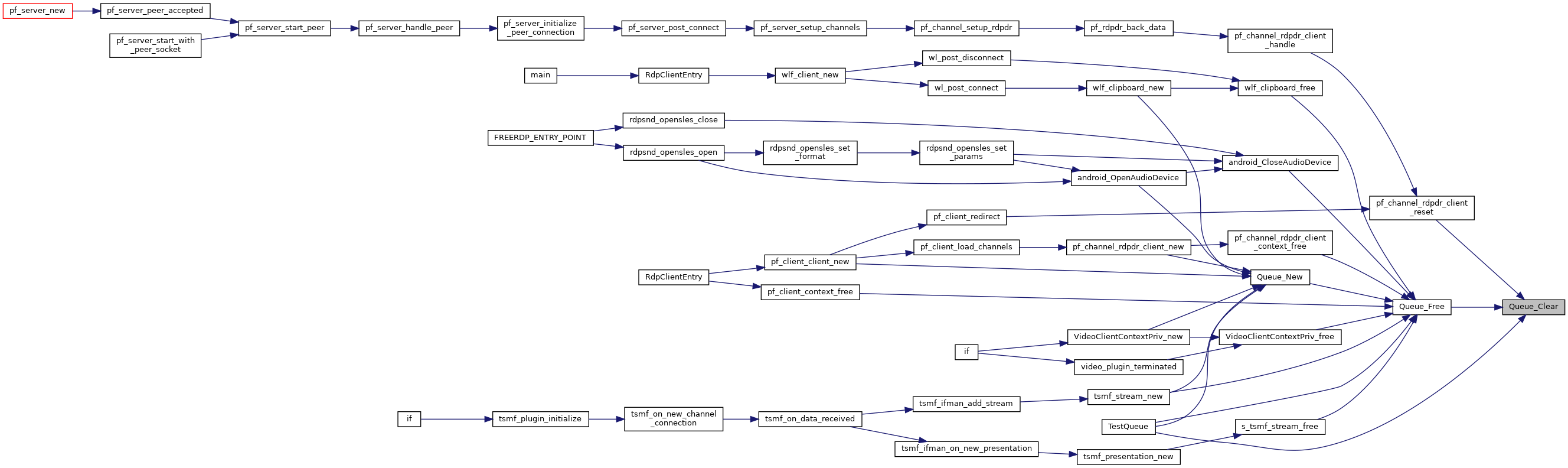## ◆ Queue_Contains()

 BOOL Queue_Contains ( wQueue * queue, const void * obj )

Determines whether an element is in the Queue.

Here is the call graph for this function: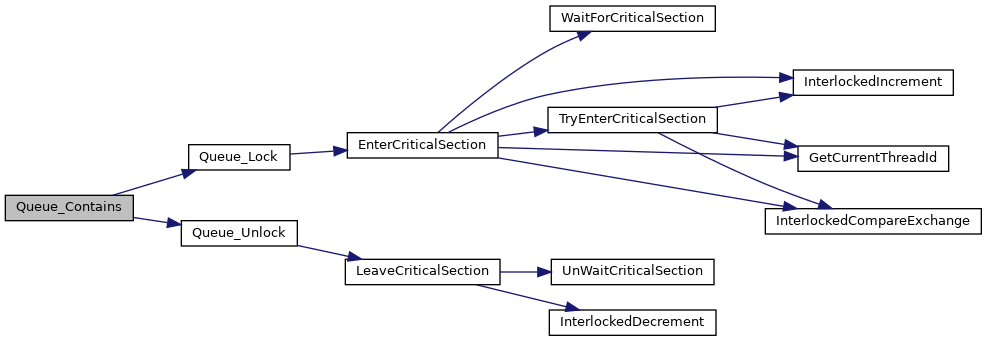## ◆ Queue_Count()

 size_t Queue_Count ( wQueue * queue )

C equivalent of the C# Queue Class: http://msdn.microsoft.com/en-us/library/system.collections.queue.aspx Properties Gets the number of elements contained in the Queue.

Here is the call graph for this function: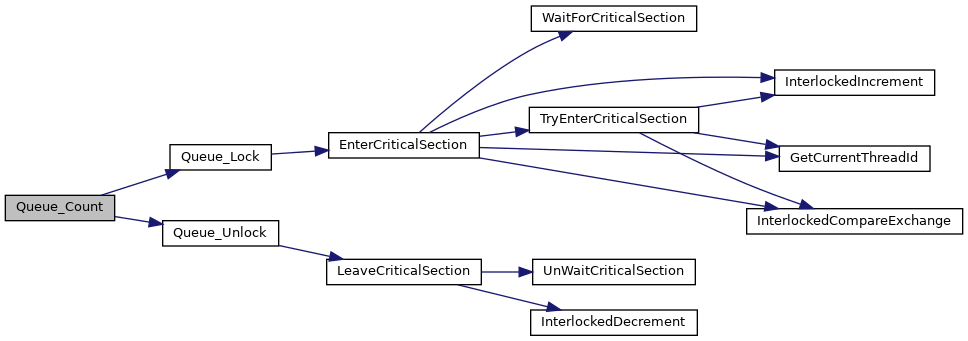Here is the caller graph for this function: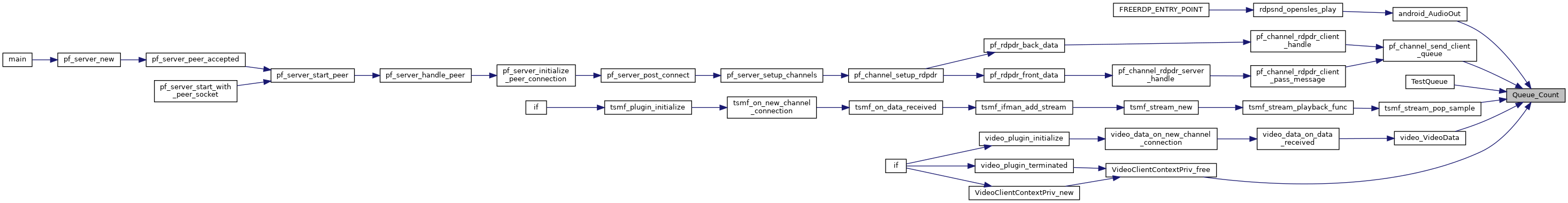## ◆ Queue_Dequeue()

 void* Queue_Dequeue ( wQueue * queue )

returns the element at the top of the queue. The element is removed from the queue, ownership of the element is passed on to the caller.

Removes and returns the object at the beginning of the Queue.

Here is the call graph for this function: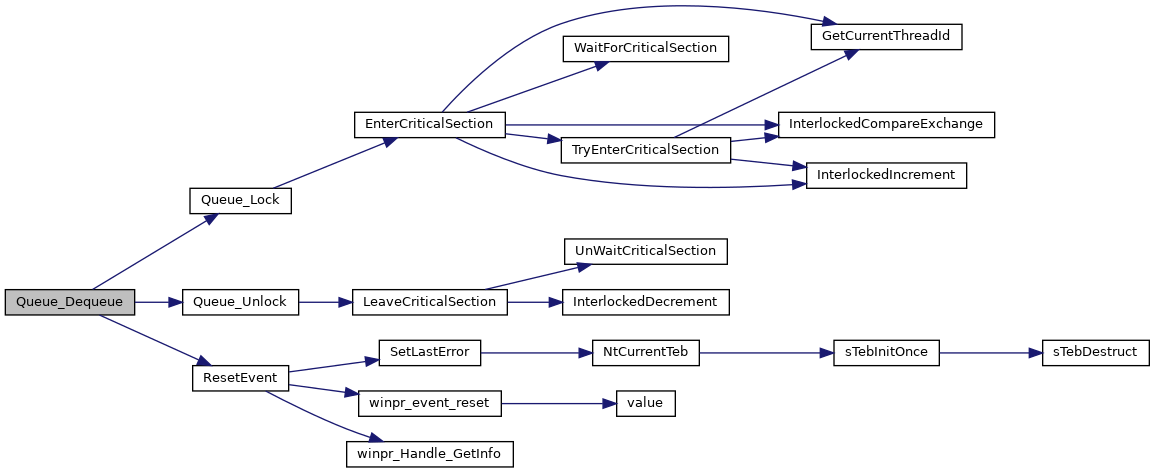Here is the caller graph for this function: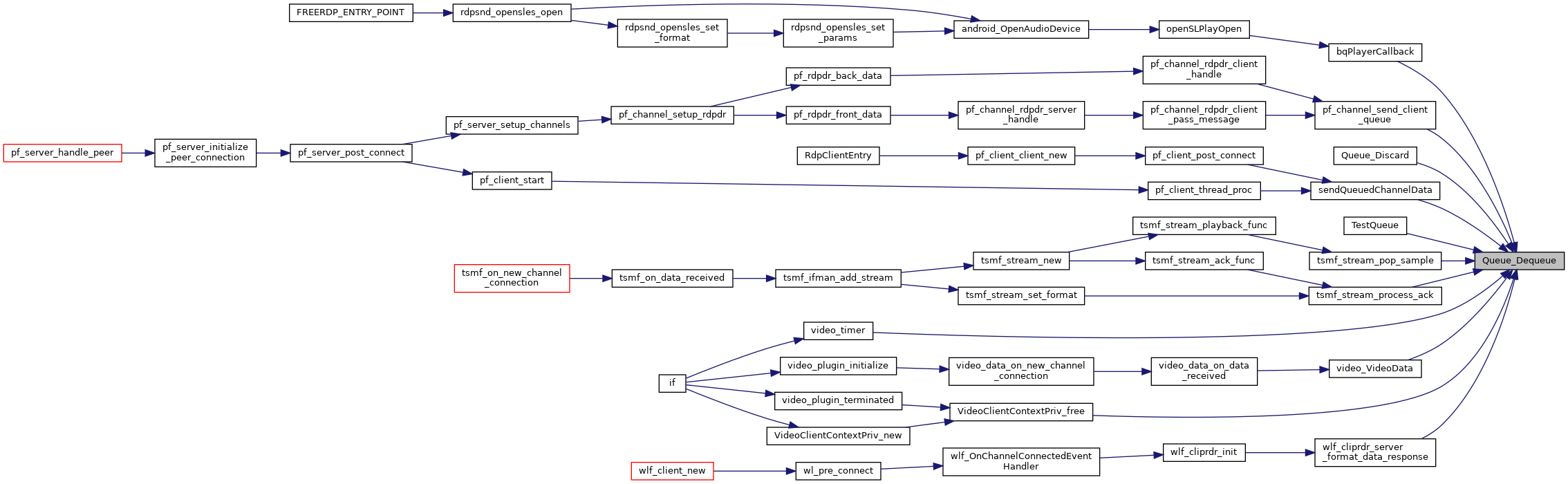void Queue_Discard ( wQueue * queue )

Removes the element at the top of the queue. If fnObjectFree is set, the element is freed. This can be used in combination with Queue_Peek to handle an element and discard it with this function afterward. An alternative is Queue_Dequeue with calling the appropriate free function afterward.

Parameters
 queue The queue to operate on
Here is the call graph for this function: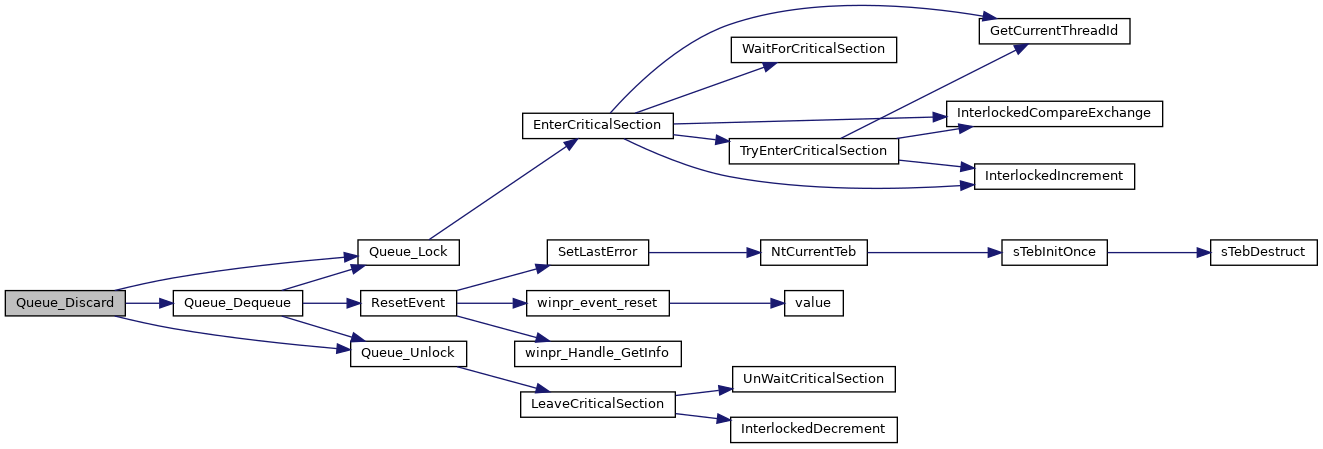## ◆ Queue_Enqueue()

 BOOL Queue_Enqueue ( wQueue * queue, const void * obj )

Pushes a new element into the queue. If a fnObjectNew is set, the element is copied and the queue takes ownership of the memory, otherwise the ownership stays with the caller.

Adds an object to the end of the Queue.

Here is the call graph for this function: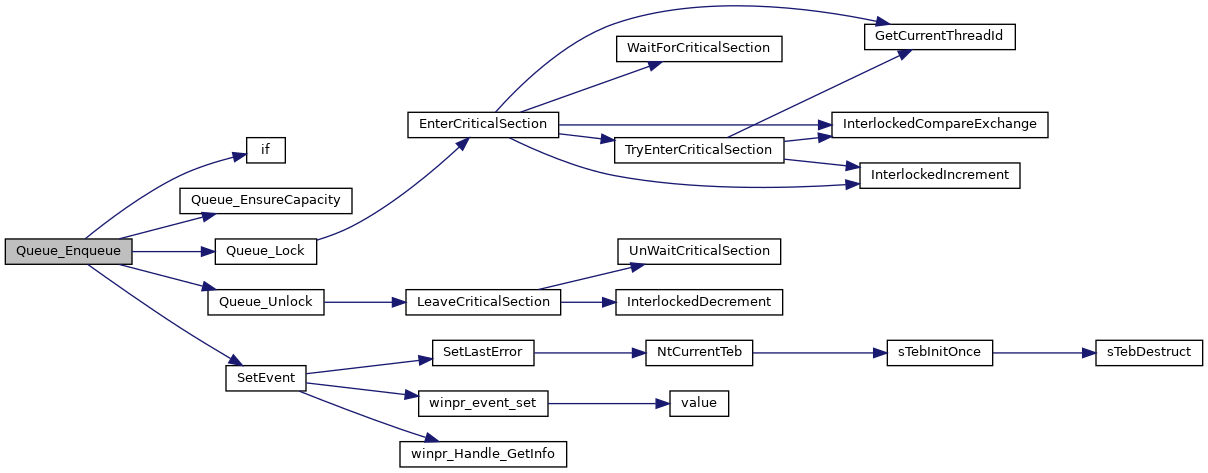Here is the caller graph for this function: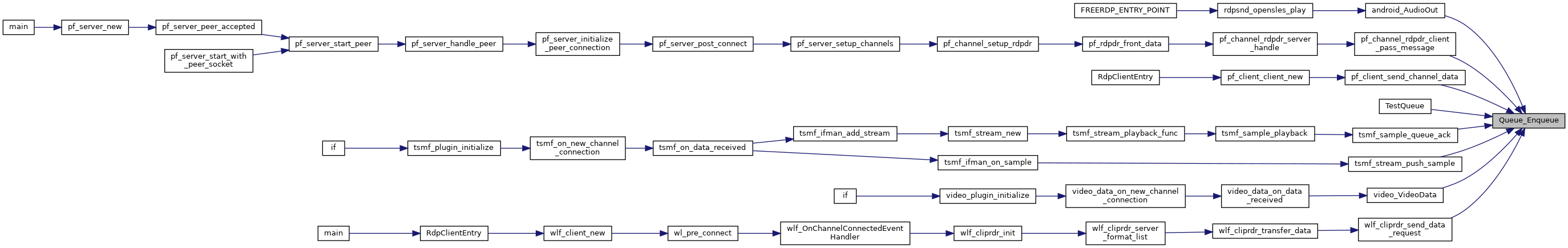## ◆ Queue_EnsureCapacity()

 static BOOL Queue_EnsureCapacity ( wQueue * queue, size_t count )
static
Here is the caller graph for this function: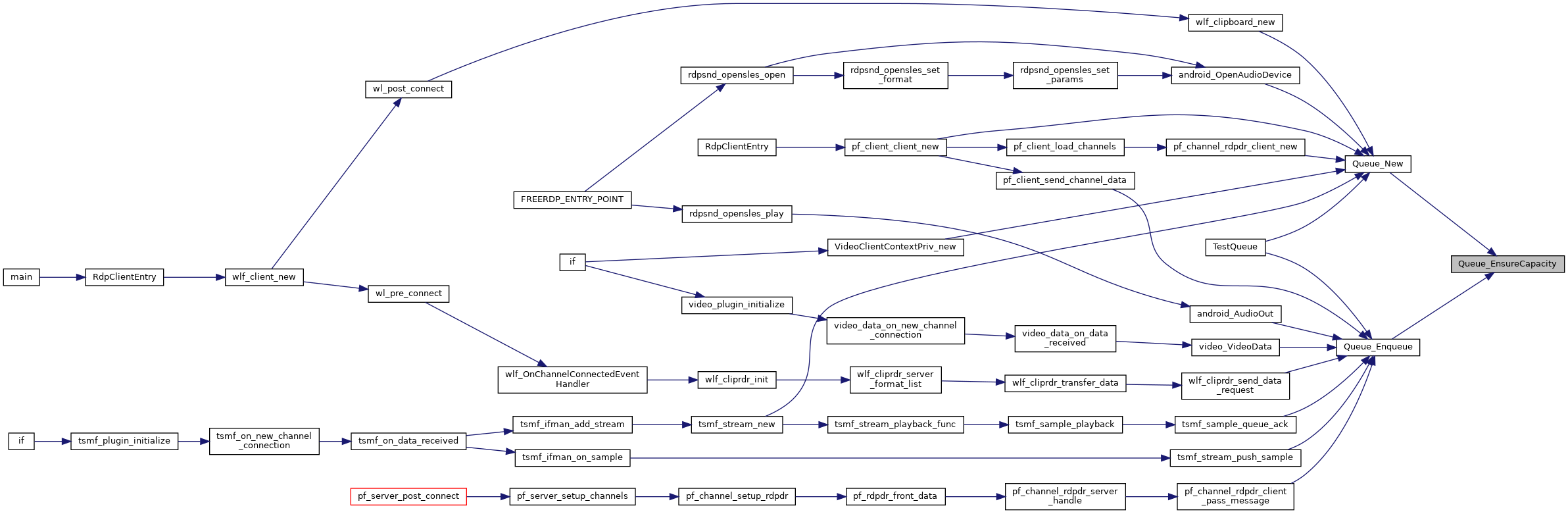## ◆ Queue_Event()

 HANDLE Queue_Event ( wQueue * queue )

Gets an event which is set when the queue is non-empty

Here is the caller graph for this function: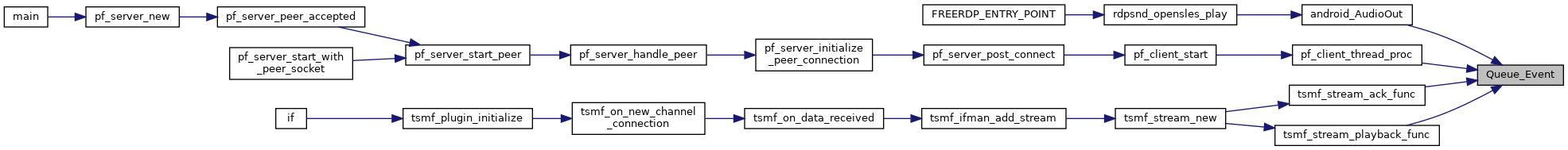## ◆ Queue_Free()

 void Queue_Free ( wQueue * queue )
Here is the call graph for this function: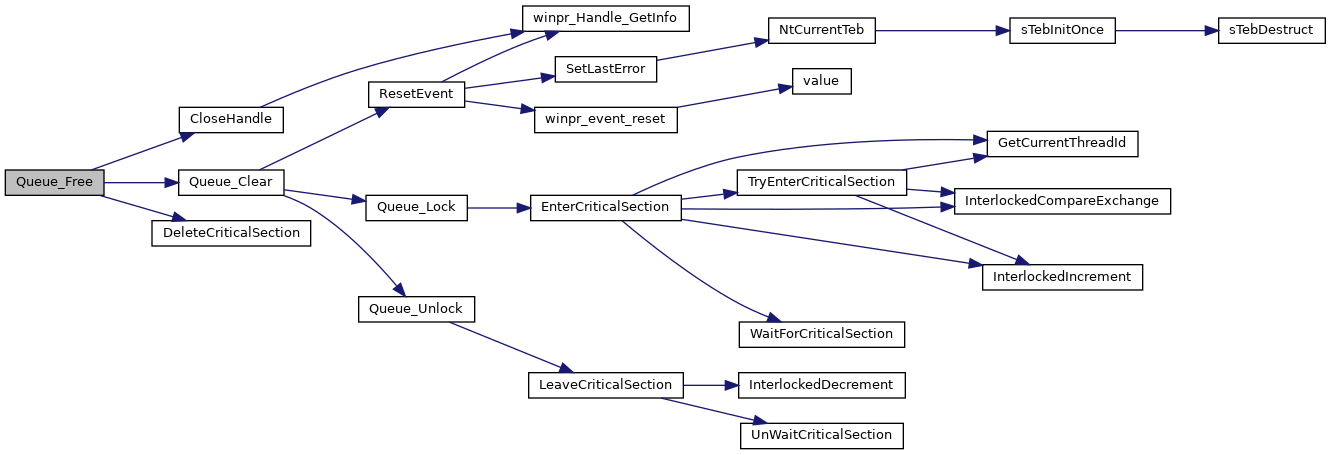Here is the caller graph for this function: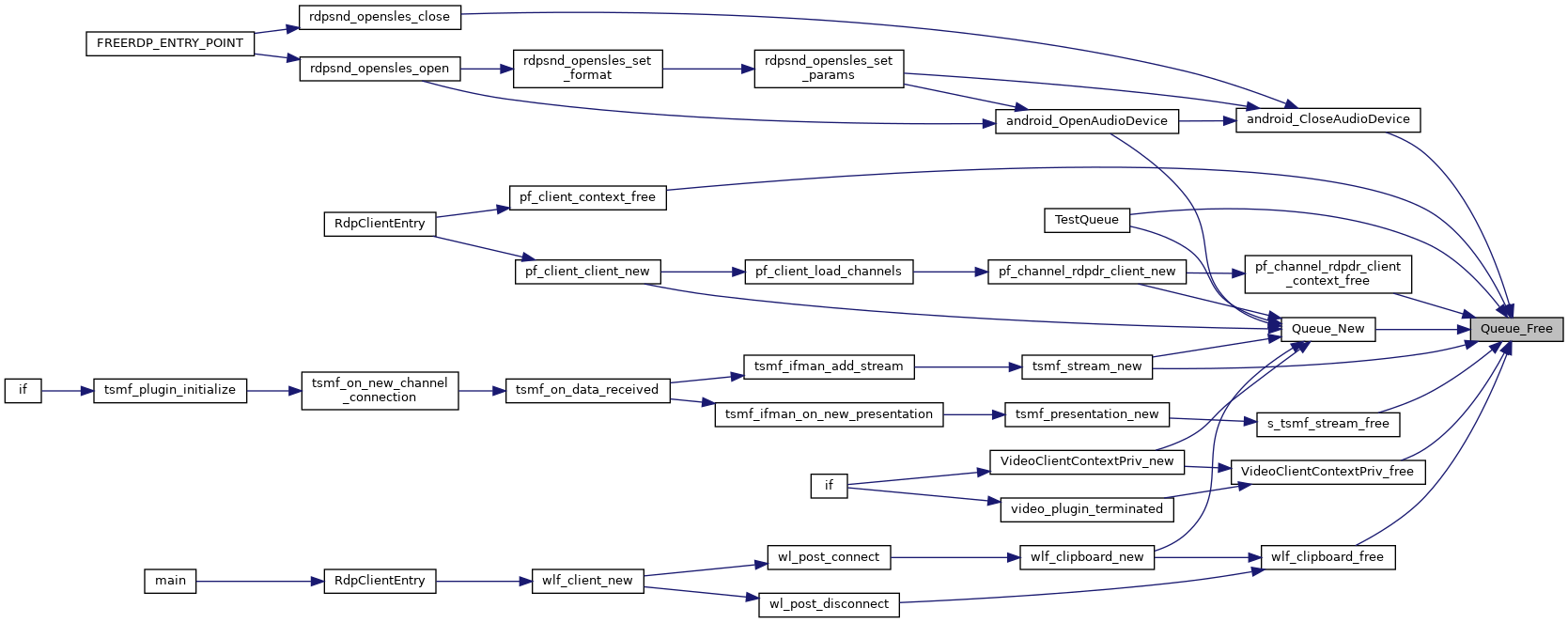## ◆ Queue_Lock()

 void Queue_Lock ( wQueue * queue )

Here is the call graph for this function: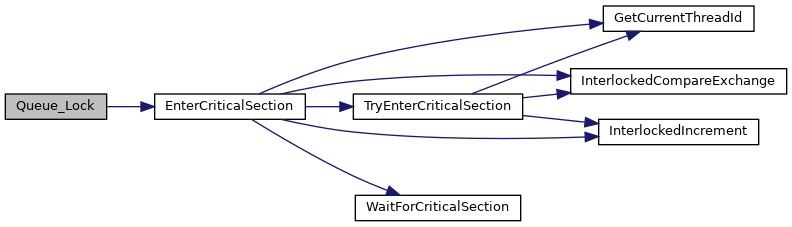Here is the caller graph for this function: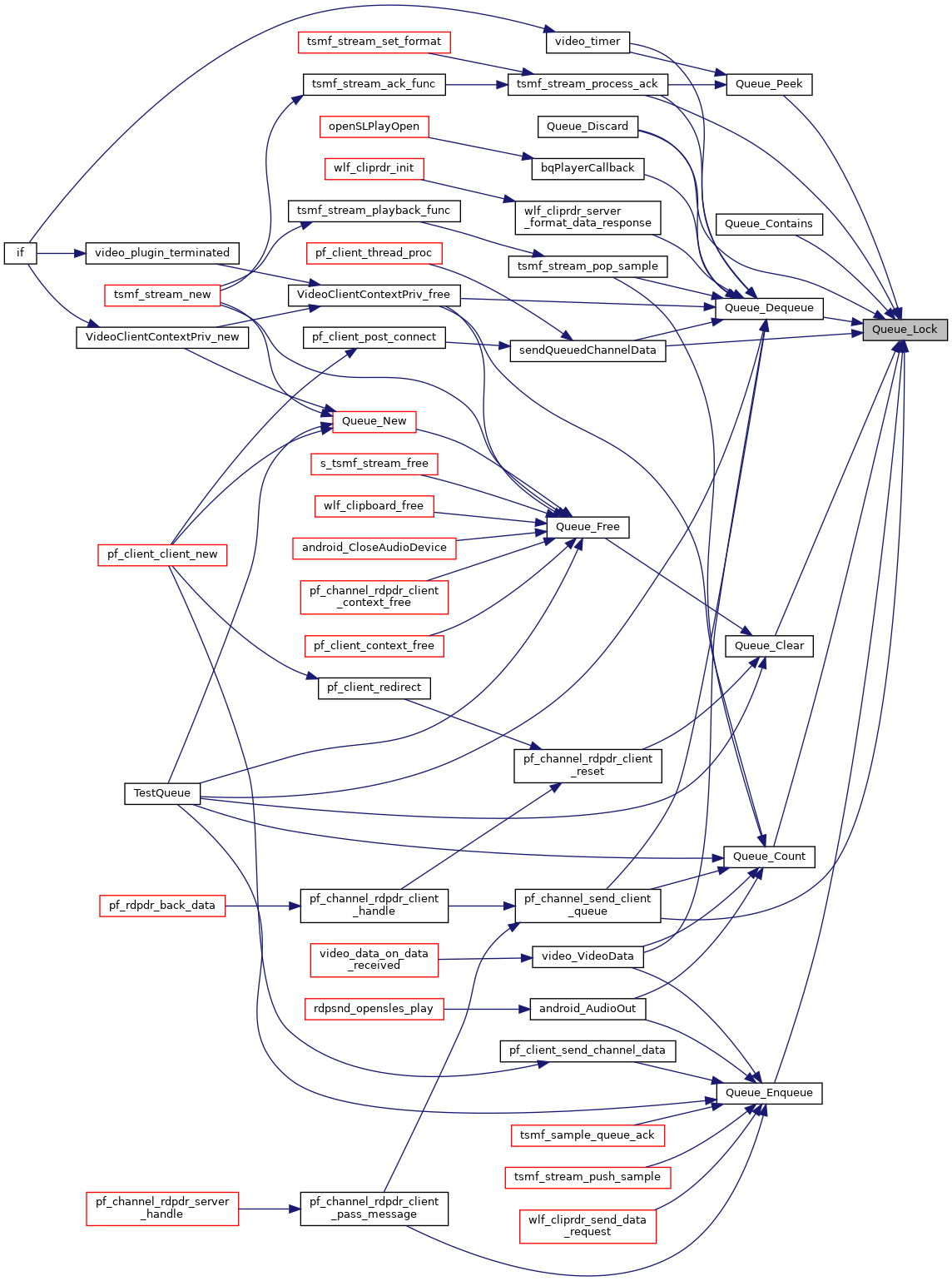## ◆ Queue_New()

 wQueue* Queue_New ( BOOL synchronized, SSIZE_T capacity, SSIZE_T growthFactor )

Construction, Destruction

Here is the call graph for this function: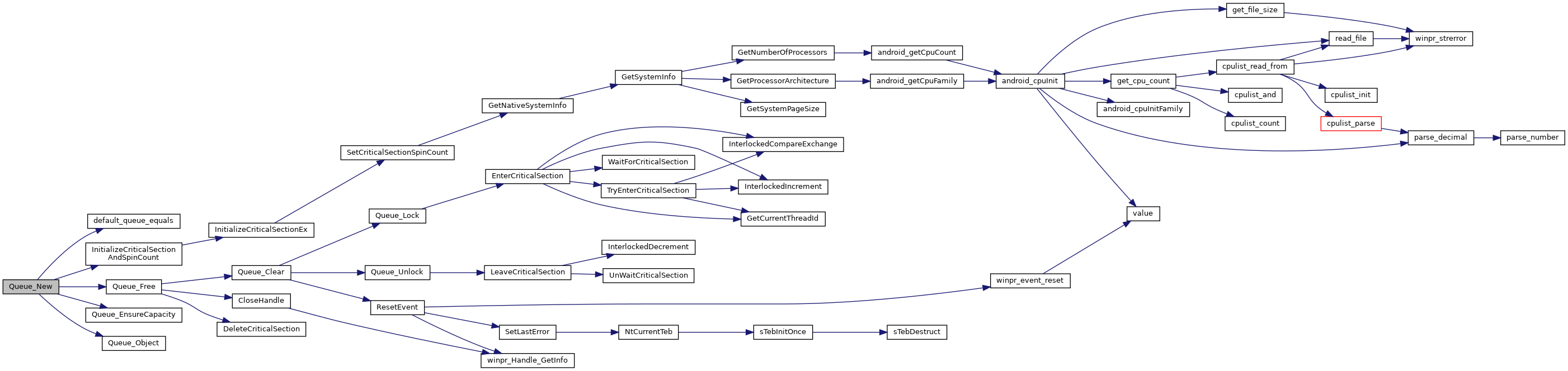Here is the caller graph for this function: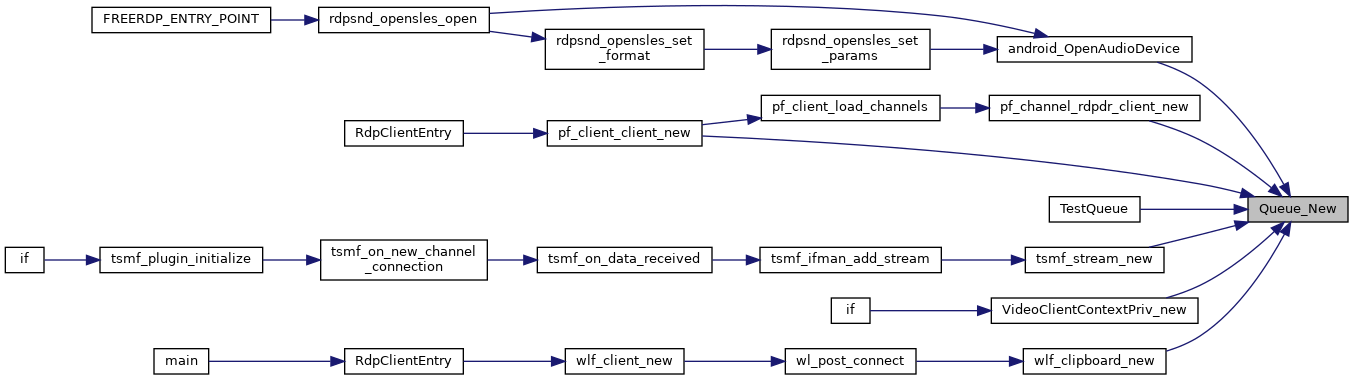## ◆ Queue_Object()

 wObject* Queue_Object ( wQueue * queue )
Here is the caller graph for this function: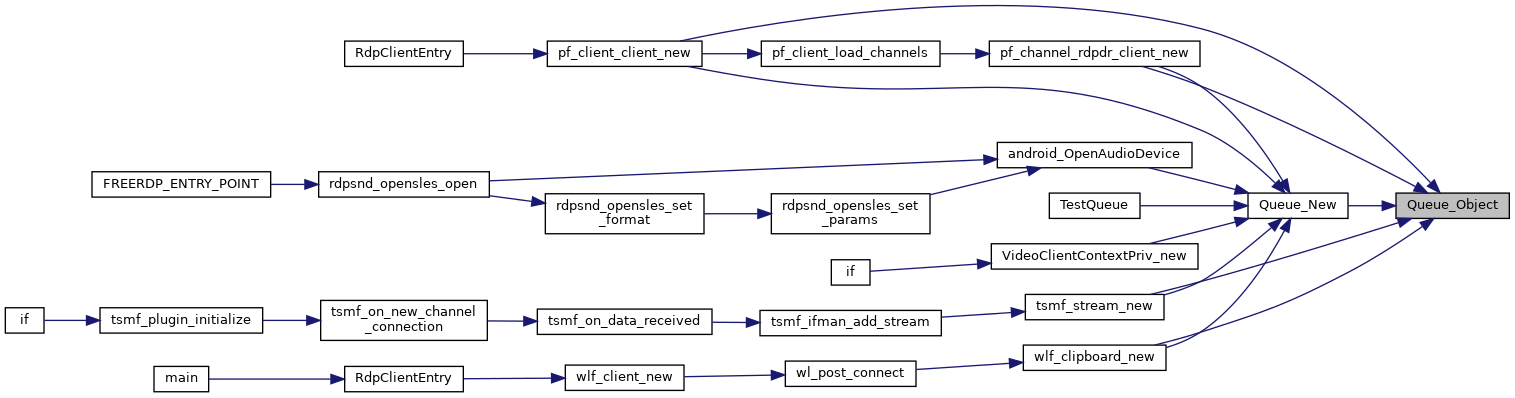## ◆ Queue_Peek()

 void* Queue_Peek ( wQueue * queue )

returns the element at the top of the queue. The element is not removed from the queue, ownership of the element stays with the queue.

Returns the object at the beginning of the Queue without removing it.

Here is the call graph for this function: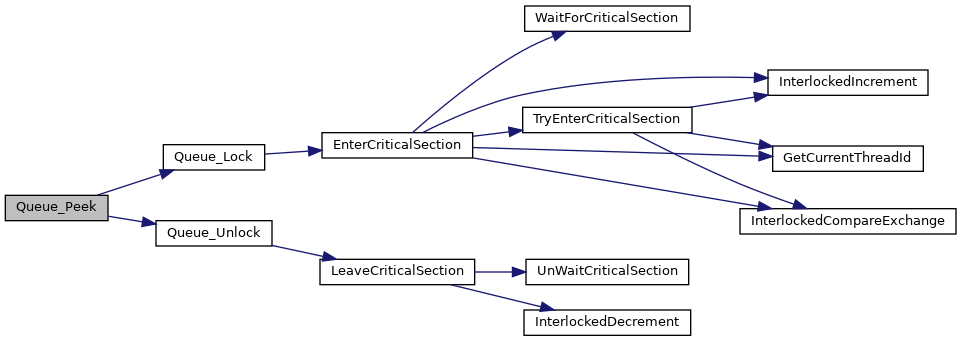Here is the caller graph for this function: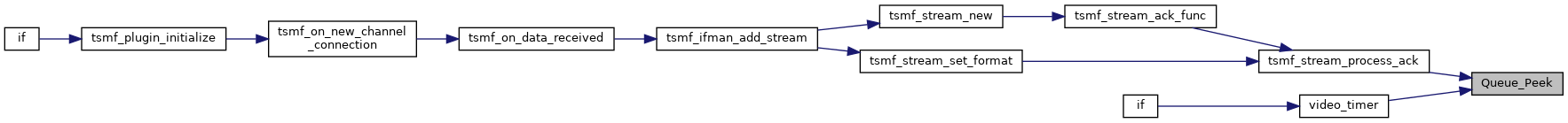## ◆ Queue_Unlock()

 void Queue_Unlock ( wQueue * queue )